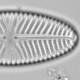I needed some temperature data from Poland for some work I am doing on the pheonology of understorey plants in Białowieża Forest.

The easy source of data is the GHCN which can be accessed through the `rnoaa` package. This code loads the necessary packages and finds stations in GHCN within 200 km of Białowieża Forest.

```#Download Bialowieza and regional temperature data
library("tidyverse")
library("rnoaa")
library("lubridate")
library("ggrepel")

##regional climate
#find nearby sites
longitude, latitude, id
23.894614, 52.744313,Białowieża"
)

stations <- meteo_nearby_stations(dat, lat_colname = "latitude", lon_colname = "longitude", station_data = ghcnd_stations(), var = "all",  year_min = 1960, year_max = 2000, radius = 200)

#map
mp <- map_data("world", xlim = c(16, 30), ylim = c(48, 58))

ggplot(stations\$Białowieża, aes(x = longitude, y = latitude, label = name)) +
geom_map(data = mp, mapping = aes(map_id = region), map = mp, fill = "grey80", colour = "black", inherit.aes = FALSE) +
geom_point() +
geom_label_repel() +
geom_point(data = dat, aes(x = longitude, y = latitude), colour = "red", size = 3, inherit.aes = FALSE)
```Climate stations near Bialowieza Forest (red dot)

```#download data
regionalData <- stations\$Białowieża %>%
filter(distance < 70) %>% # 100 km radius
group_by(name, id) %>%
mutate(tavg = tavg/10,
variable = "tavg") %>%
rename(value = tavg)

#plot regional data
g <- regionalData %>% filter(year(date) == 2000) %>%
ggplot(aes(x = date, y = value, colour = name, group = name)) +
geom_line() +
labs(x = "Date", y = "Daily mean temperature °C", colour = "Station")
print(g)

```Mean daily temperature for the year 2000 for the four nearest GHCN stations

Unfortunately, only a small proportion of the Polish weather data are available through GHCN. Until last year, these data were difficult to access. Now they are available to download from https://dane.imgw.pl/.

It is only possible to download seven days’ data at once. This would become tedious if you wanted data for several years. I wanted 50 years’ data, so I wrote an R function to hit the server ~2500 times slowly (server limit of 1000 queries per client per ten minutes). To use the script, you need to know the site ID for the weather station (map), the variable name (hope your Polish is good), and a registration. What I haven’t found are meta-data showing which stations have which variables and for how long.

```## Bialowieza data from
source("R/get_polish_weather.R")

#save(authentic, file = "data/authentic.Rdata")
startDate <- as.Date("2000-1-1")
endDate <- as.Date("2000-12-31")
siteCode <- "252230120"

siteCode = siteCode,
variableCode = "B100B007CD",
startDate = startDate,
endDate = endDate,
authentic = authentic
)

mutate(name = "Białowieża", variable = "tavg")
```

And a plot to compare the Białowieża data with the GHCN data

```## compare Białowieża with regional data

g %+% filter(all_temperatures, year(date) == 2000)
```The four nearest GHCN stations and Bialowieza

Not surprisingly, all the data are in good agreement, but note that the GHCN-daily data are not homogenised to account for station moves etc. I suspect the IMGW data are not homogenised either.1.richard telford says: